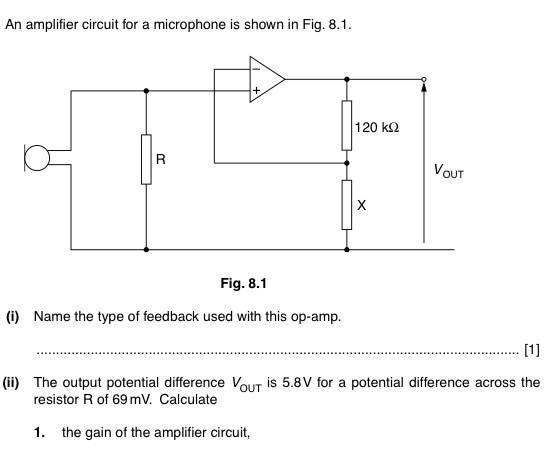# Opamp problem

1. Homework StatementFor part ii) ,
V+ is 69mV, but how to determine V-? We don't know the resistance of X, so we can't find the p.d. across X, so how to find V-?

## Homework Equations

Vout =A0(V+-V-)

gleem
Isn't the gain of this amp Vout/VR?

•CWatters
gleem
But Vout and VR are given.

•CWatters
I'm sorry, but can you please explain to me why is V-=0 in your eqn for the gain in post #2 , thanks

Last edited by a moderator:
gleem
There is no zero in my equation in post #2

Last edited by a moderator:
There is no zero in my equation in post #2
Then, how did you know that the gain is Vout/VR? Why didn't you take account of V-?

Last edited by a moderator:
gleem
That is the general definition of gain.

OK.
Vout=Ao(V+-V-), am I right?
Vout=5.8V
V+=69mV
V-= ?

gleem
Why do you want V- ?

Why do you want V- ?
So that I can use the formula, Vout=A0(V+-V-) ,and get A0

gleem
The question was what is the gain of the circuit not the op-amp. that's why I commented on the simplicity of the question.

So that I can use the formula, Vout=A0(V+-V-) ,and get A0
So you are interested in Ao ???
Up to now I was of the opinion you were looking for the gain of the whole amplifier stage?

NascentOxygen
Staff Emeritus